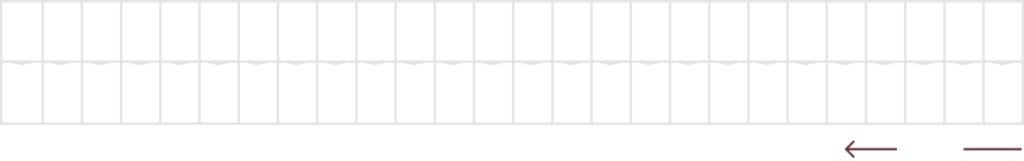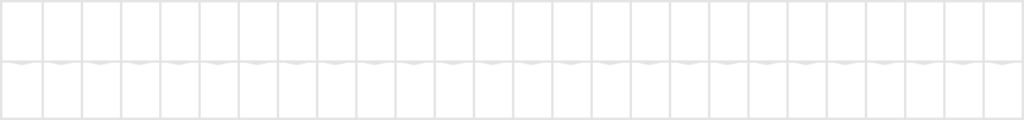Back To All

# The Shift CipherThe shift cipher, or the better-known name the Caesar cipher named after Julius Caesar is one of the simplest encryption techniques. In fact for those who have read my Substitution cipher post, the shift cipher is a type of substitution cipher where each letter in the alphabet is mapped to another fixed letter in the alphabet. But you do not have to of read the Substitution cipher post to understand this post.

But it is highly recommended to read the last Introduction to Modular Arithmetic and Equivalence classes post to understand the math behind the shift cipher. The Shift cipher will also be the basis for another cipher covered latter, the Vigenere cipher so if you’re interested in that be sure to go check that out after reading this post first.

## Shift Cipher

The idea behind the shift cipher is to shift each letter in the alphabet by a fixed amount, this shift will be our key and you can choose an amount within ℤ26  ie from 0 – 25. Here is example diagram of that mapping with a shift of k = 5:Notice the wrap-around for V, W, X, Y, Z. This is done very nicely with modulo 26 arithmetic if we just encoded each letter with a number. So lets do exactly that and give each letter a number from 0 – 25 so that we can do modular arithmetic on them.Now that we have an integer representation of each letter in the alphabet we can create formulas for our encryption and decryption.

### Algorithm

Let x , y, k ∈ ℤ26
Encryption: ek(x) ≡ x + k mod 26
Decryption: dk(y) ≡ y – k mod 26

### Example

Let k = 16, and the plaintext is “ATTACK”
First encoding our letters as numbers:
ATTACK = 0,19,19,0,2,10
Now using our cipher:
0,19,19,0,2,10 = 16, 9, 9, 16, 18, 9 = QJJQSA

### Code Implementation

The Shift cipher is very easy to implement in code once we understand modular arithmetic, you may notice that the coded algorithm looks a little different. That is because in code letters already have different encoded numbers called ASCII, but it works exactly the same.

```				```
#include <iostream>
#include <string>

using namespace std;

string encrypt(string plaintext, string ciphertext, int plaintextSize, int k);

int main()
{
// enter plaintext and decide a shift amount
string plaintext = "attack";
int k = 16;

// get length of plaintext for loop
int plaintextSize = plaintext.length();

string ciphertext = "";
ciphertext = encrypt(plaintext, ciphertext, plaintextSize, k);

cout << "Encrypted Ciphertext:  " << ciphertext << endl;
}

string encrypt(string plaintext, string ciphertext, int plaintextSize, int k)
{
for (int i = 0; i < plaintextSize; i++)
{
// use ASCII value of letter to normalize and shift using modular arithmetic
ciphertext += char(int(plaintext[i] + k - 97) % 26 + 97);
}

return ciphertext;
}
```
```

### Attacks

As you can guess after the discussion in the substitution cipher, the shift cipher is not secure at all. There are two main ways of attacking it:
Brute-Force: Since there are only 26 unique keys (shift positions) you can easily even by hand try all 26 keys. You know you found your answer when the text is readable, thus you also found the key.
Letter Frequency-Analysis: As in the substitution cipher, the shift cipher has the same weakness, which is its fixed mapping. Therefore, this attack also works against the shift cipher.

If you want to check out the brute-force attack in code make sure to check out my GitHub here.

## Affine Cipher

The affine cipher is a very similar cipher to the shift cipher, and trys to improve it by increasing the number of keys. Instead of just adding the key, we will now multiply with one part of the key, and add with another part of the key.

### Algorithm

Define k = (a, b). Let x , y, a, b ∈ ℤ26 i
Encryption: ek(x) ≡ a ⋅x + b mod 26
Decryption: dk(y) ≡ a-1 (y – b) mod 26
Note the restriction that gcd(a, 26) = 1.

The restriction is important because if you remember in the Introduction to Modular Arithmetic and Equivalence classes post, not all elements in the integer ring have a multiplicative inverse.

### Example

Let k = (a, b) = (21, 7), and the plaintext is “ATTACK”
First encoding our letters as numbers:
ATTACK = 0,19,19,0,2,10
Now using our cipher:
0,19,19,0,2,10 = 7, 16, 16, 7, 23, 8 = HQQHXJ

### Code Implementation

The affine cipher is very easy to implement in code once we understand modular arithmetic, you may notice that the coded algorithm looks a little different. That is because in code letters already have different encoded numbers called ASCII, but it works exactly the same.

```				```
#include <iostream>
#include <string>

using namespace std;

string encrypt(string plaintext, string ciphertext, int plaintextSize, int a, int b);

int main()
{
// enter plaintext and decide a shift amount
string plaintext = "attack";
int a = 21;
int b = 7;

// get length of plaintext for loop
int plaintextSize = plaintext.length();

string ciphertext = "";
ciphertext = encrypt(plaintext, ciphertext, plaintextSize, a, b);

cout << "Encrypted Ciphertext:  " << ciphertext << endl;
}

string encrypt(string plaintext, string ciphertext, int plaintextSize, int a, int b)
{
for (int i = 0; i < plaintextSize; i++)
{
// use ASCII value of letter to normalize and shift using modular arithmetic
ciphertext += char(((a * int(plaintext[i] - 97)) + b) % 26 + 97);
}

return ciphertext;
}
```
```

### Attacks

Is the affine cipher secure? No, the key space even though improved is still not big enough to be considered secure. The key space for the affine cipher is:
|k| = (#values of a) x (#values of b) = 12 x 26 = 312
A key space of only 312 while probably tedious if done by hand can still be done, and even trivial for a computer to do it. So again as the shift cipher here are two attacks that work against the affine cipher:
Brute-Force: Since there are only 312 unique keys (shift positions) you can get a computer to find all decrypted plaintext. You know you found your answer when the text is readable, thus you also found the key.
Letter Frequency-Analysis: As in the shift cipher and substitution cipher, the affine cipher has the same weakness, which is it still has a fixed mapping. Therefore, this attack also works against the shift cipher.